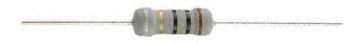﻿ Parts Per Million (PPM)- Explained ﻿Parts Per Million (PPM)- ExplainedParts Per Million (PPM) is a method of stating a component's tolerance value by specifying how many parts per million units a component may vary from its nominal value.

This will all be explained below.

Ways of Specifying Electronic Component Tolerance

The tolerance of electronic components can be stated in one of 2 ways. Probably the most common way of specifying an electronic component's tolerance is by percentage. For example, a 10KΩ having a tolerance of 20%. This means that the resistance may vary by 20% of this nominal value. Since 20% of 10KΩ is 2000, the resistance of the resistor can be between 8000Ω and 12,000Ω. So percentage is one way of specifying the tolerance of an electronic component.

The other way of specifying the tolerance is through the parts per million (ppm) specification. This is a specification which states how many parts a component may vary per million units. So when dealing with resistance, it shows how many ohms of resistance a resistor may vary per million ohms of resistance. If dealing with capacitance, it shows how many farads a capacitor may vary per million farads of capacitance.

Examples

Parts per million will be explained below through the following examples:

Let's say we a 1MΩ resistor.

A 1MΩ resistor is a resistor that has a color code of brown-black-green. It has a nominal value of 1,000,000Ω.

Now let's say this resistor has a parts per million specification of 5000ppm. This means that for every 1,000,000 ohms of resistance that this resistor has, the resistance can vary as much as 5000 ohms. This means that this 1MΩ can have a resistance 5000 ohms below or above 1,000,000 ohms, which means its resistance can be as low as 995,000Ω or as high as 1,005,000Ω or somewhere in between.

As another example, let's take a 15MΩ resistor which has a parts per million specification of 2000ppm. This means that for every 1 million ohms of resistance, the resistor may vary by 2000Ω. Since the resistor we are discussing has a value of 15MΩ, this means that its resistance may vary by 30,000Ω, since 15,000,000/1,000,000=15 and 15 * 2000= 30,000. So, in total, the resistor has a tolerance of 30,000Ω. This means that its resistance can vary as much as 30,000Ω above or below its nominal value of 15MΩ.

As one last example for a resistor, let's take a resistor of 10KΩ which has a parts per million specification of 2000ppm. A 10KΩ resistor does not have a million ohms of resistance. However, the parts per million specification still applies. Even though it doesn't have a million ohms of resistance, we still apply this principle by dividing the nominal value, which in this case is 10KΩ, by 1,000,000. We then take the quotient of this division and multiply it by the ppm specified value. So with the 10KΩ resistor, the calculation goes as follow, 10KΩ/1,000,000= 0.01 * 2000= 20Ω. This means that the 10KΩ resistor can vary as much as 20Ω above or below its nominal value of 10KΩ. So its resistance will be betweeen 9980Ω and 1020Ω.

And this is what parts per million represents. For every 1 million units, a component may vary by its specified ppm value. If dealing with resistance, for every 1,000,000 ohms of resistance, a resistor may vary by its ppm specified value.

Parts per million do not only apply to resistors but do also for capacitors. The tolerance of capacitors many times are also specified according to the ppm specification as well. Some types of capacitors have far superior ppm ratings than others, showing they have a tighter tolerance range, which shows they are more precise. Where precision is very important, such as in timing circuits, capacitors with low PPM ratings are used. Generally, the lower the PPM rating, the more desirable the capacitor, since it will be closer to its nominal value. However, where precision is not absolutely necessary, capacitors may not need have to adhere to low ppm ratings.

Related Resources

Parts Per Million (PPM) Calculator

PPM to Percent Calculator

Percent to PPM Calculator